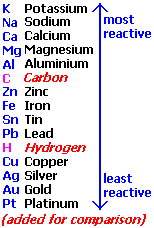# *chapter 8* chemistry – FlashcardsIsabella Parker

## Unlock all answers in this set

question
Structure of chemical equations
1. Reactants and products are separated by an arrow.Reactants are on the left side of the arrow, products are on the right. a A + b B -----> c C +d D 2.Whole number coefficients are placed in front of substances to balance the atoms in the equation. The numbers indicate the units of the substance reacted or formed during the reaction. 3.Information about the reaction may be placed above or below the reaction arrow. a A +b B -----> cC + dD 4.The physical state is written in brackets after the formula of the substance . (g) gas (l) Liquid (s) soild (aq) for aqueous aA (s) + bB (l)----->cC (g) + dD(s)
question
Law of conservation of mass
The total mass of substances in a chemical reaction must remain constant. Water ---> hydrogen +oxygen 100.0g 11.2 g 88.8 Reactants 100.0g total of products
question
writing & balancing chemical equations
contain the same number of each kind of atom on both sides of the equation. 1.identify the reaction write a word equation for the reaction mercury (II) oxide -----> mercury + oxygen 2.write the unbalanced (skeleton) equation. write the correct formula for each substance HgO------> Hg + O2 3. Balance the equation. a. count the number of each atom on the reactants and products side and determine what requires balancing. HgO ---> Hg + O2 Hg:1 Hg:1 O: 1 O:2 Oxygen atoms need balancing on the reactants side.
question
reactants
substances consumed during the reaction.
question
Products
substances formed during the reaction.
question
Balanced equation
contains the same number of each kind of atom on each side of the equation. obeys the law of conservation of mass. 1. identify the reaction. With a description or word equation for the reaction. EX: Mercury (II) oxide decomposing into mercury and oxygen. mercury (II) oxide ---> mercury + oxygen 2. write the unbalanced equation. make sure that the formula for each substance is correct and that reactants are written to the left and products to the right of the arrow. Ago--> hg + O 2 3. balance the equation. use the following process as necessary: a) count & compare the number of atoms of each element on each side of the equation and determine those that must be balanced Hg is balanced ( 1 on each side) O needs to be balanced (1 on reactant side, 2 on product side) b)balance each element one at a time, by placing whole numbers ...
question
polyatomic ion
an ion composed of more than one atom.
question
Information in a Chemical Equation
propane, oxygen ,carbon dioxide, water. (look at pic )
question
Reactions are classified into five major categories:
1. Combination reactions 2. Decomposition reactions 3. Single displacement reactions 4. Double displacement reactions 5. Oxidation-reduction (redox) reactions (Chapter 17)
question
Combination Reactions
Two reactants combine to give a single product. A + B-----> AB where A or B are elements/compounds and AB is a compound.
question
Decomposition Reactions
A single reactant breaks down (decomposes) into two or more products . AB ------> A + B Representative Examples 2 PbO2 (s) Δ 2 PbO(s) + O2 (g)
question
Single Displacement Reactions
One element (A) reacts with a compound (BC) to replace one element in the compound, giving a new element (B) and a different compound (AC). A + BC------> B + AC
question
Activity SeriesTable listing in order of decreasing reactivity of various elements. More reactive elements replace less reactive ones. 2 Al (s) + 3 CuCl2 (s) 2 AlCl3 (aq) + 3 Cu (s) 2 Ag (s) + 3 CuSO4 (s) No Reaction
question
Double Displacement Reactions
Two compounds exchange partners with one another to yield two new compounds. AB + CD ------> AD + CB Physical evidence for a double displacement reaction include: heat evolution, formation of a precipitate or gas production.
question
Exothermic reactions: